# Stoichiometry Tutorials: Dimensional Analysis / Stoichiometric Conversions

(from a complete OLI stoichiometry course)

Dimensional analysis allows us to change the units used to express a value. For instance, it allows us to convert between volume expressed in liters and volume expressed in gallons. The following video gives a brief overview of dimensional analysis, including conversion between the amount of a substance expressed in "number of molecules" and the amount of a substance expressed in "moles of molecules."

### Dimensional Analysis Movie Text

Stoichiometry provides a set of tools that chemists use to manipulate quantities of substances. In working with chemical quantities, it is important to remember that each quantity is associated with both a unit and a chemical substance, and it is important to always write both of these down. For example, we will write 4.1 kg water, or 0.23 mol oxygen, or 3.0 x 1021 atoms sodium.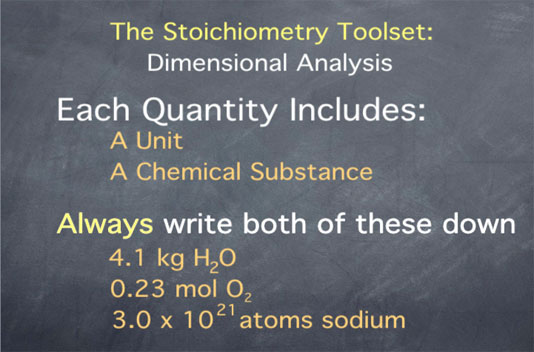Dimensional analysis provides us with the tools needed to convert between different units of measure.

Let's say we have the definition "one kilogram is equal to 1000 grams".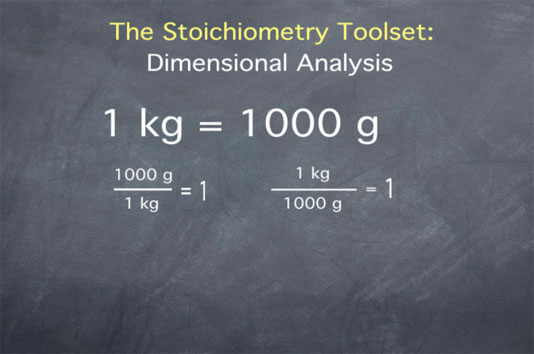We can take this definition and form ratios:

• 1000 grams over 1 kilogram is equal to 1.
• 1 kilogram over 1000 grams is equal to 1.

These ratios are useful, since they allow us to convert from quantities in grams to quantities in kilograms and vice versa.

Consider, for example, the quantity 4.1 kilograms of water. Say we want to convert this quantity to grams of water. We begin by writing down our initial quantity of 4.1 kilograms water. Next we multiply by the ratio 1000 grams of water per 1 kilogram water. Remember that 1000 g and 1 kg are the same thing, so we are just multiplying our initial quantity by 1. This multiplication does not change the amount of water; it merely changes the units we are using to describe this amount of water.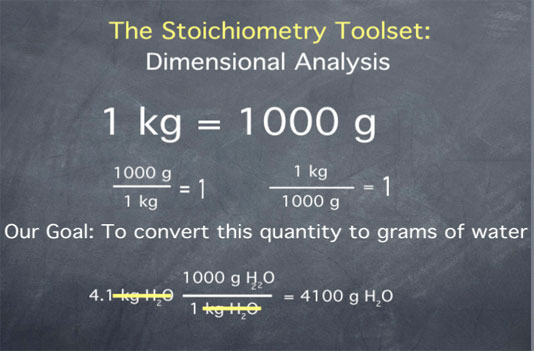After multiplying, we get the value 4100. To determine the units of this quantity, we cancel the kilograms water and are left with grams water. Therefore, we have achieved our goal of converting the quantity "4.1 kilograms of water" to that same amount expressed in "grams of water".

Let's do another example of a unit conversion. Say we are given the density of water as one gram of water per milliliter of water, and we want to express this in units of grams of water per liter of water. For this conversion, we will need the definition that 1 liter is equal to 1000 milliliters.

We begin by writing our initial quantity. Our goal is to convert the units of the denominator from milliliters of water to liters of water.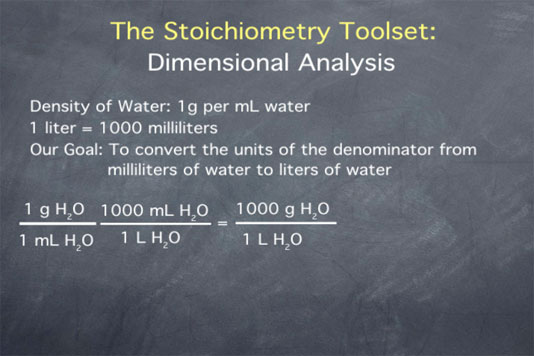We can do this by multiplying by the ratio 1000 milliliters of water over 1 liter of water. From the above definition, we know this ratio is equal to 1, so we are changing only the unit of the quantity, not the quantity itself. The multiplication gives a value of one thousand and units of grams of water per liter of water, so we have successfully converted the density of water from units of grams per milliliter to units of grams per liter.

A particularly useful unit in chemistry is that of the mole. The mole is defined as Avogadro's number of items, where Avogadro's number (often abbreviated as NA) has the value 6.02 x 1023.

The definition of the mole can be written as one mole equals 6.02 x 1023 items. This unit definition gives us the ratios

• 1 mole over Avogadro's number of items equals 1
• Avogadro's number of items over 1 mole equals 1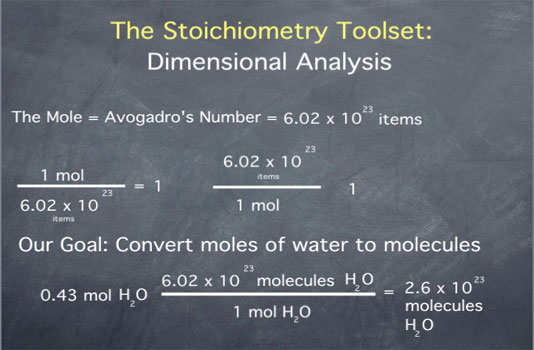Let us say that we have 0.43 mole of water, and we would like to convert this to molecules of water. We begin by writing down our initial quantity of 0.43 mol water. To convert this to molecules of water, we multiply by the ratio "Avogadro's number of water molecules per mole of water molecules". The multiplication gives the value of 2.6 x 1023, with units of molecules of water. Once again, dimensional analysis has helped us express a quantity in the units we desire.

Remember that it is always a good idea to write both the unit and substance associated with any chemical quantity; e.g., 1.3 g H2O or 5.4 x 1023 molecules H2 instead of 1.3 g or 5.4 x 1023 molecules. This will help you keep track of your quantities correctly and prevent you from making mistakes in your computations.

Conversion table for problems below.

 Mass: 1 lb = 16 oz = 453.6 g 1 kg = 1000 g = 2.20462 lb Volume: 1 gal = 3.7854 L 1 cm3 = 1mL
The content above has been converted from Adobe Flash Player and may not display correctly.